# Resources tagged with: Quadratic equations

Filter by: Content type:
Age range:
Challenge level:

There are 38 NRICH Mathematical resources connected to Quadratic equations, you may find related items under Algebraic expressions, equations and formulae.### Partly Circles

##### Age 14 to 16 Challenge Level:

What is the same and what is different about these circle questions? What connections can you make?### Interactive Number Patterns

##### Age 14 to 16 Challenge Level:

How good are you at finding the formula for a number pattern ?### In Between

##### Age 16 to 18 Challenge Level:

Can you find the solution to this algebraic inequality?##### Age 16 to 18 Challenge Level:

Find all real solutions of the equation (x^2-7x+11)^(x^2-11x+30) = 1.### Proof Sorter - Quadratic Equation

##### Age 14 to 18 Challenge Level:

This is an interactivity in which you have to sort the steps in the completion of the square into the correct order to prove the formula for the solutions of quadratic equations.### How Old Am I?

##### Age 14 to 16 Challenge Level:

In 15 years' time my age will be the square of my age 15 years ago. Can you work out my age, and when I had other special birthdays?### Always Two

##### Age 14 to 18 Challenge Level:

Find all the triples of numbers a, b, c such that each one of them plus the product of the other two is always 2.### Square Mean

##### Age 14 to 16 Challenge Level:

Is the mean of the squares of two numbers greater than, or less than, the square of their means?### Golden Thoughts

##### Age 14 to 16 Challenge Level:

Rectangle PQRS has X and Y on the edges. Triangles PQY, YRX and XSP have equal areas. Prove X and Y divide the sides of PQRS in the golden ratio.### A Third of the Area

##### Age 14 to 16 Short Challenge Level:

The area of the small square is $\frac13$ of the area of the large square. What is $\frac xy$?##### Age 11 to 14 Challenge Level:

Surprising numerical patterns can be explained using algebra and diagrams...##### Age 16 to 18 Challenge Level:

What do you get when you raise a quadratic to the power of a quadratic?### Bird-brained

##### Age 16 to 18 Challenge Level:

How many eggs should a bird lay to maximise the number of chicks that will hatch? An introduction to optimisation.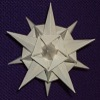### Symmetrically So

##### Age 16 to 18 Challenge Level:

Exploit the symmetry and turn this quartic into a quadratic.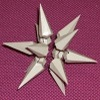##### Age 16 to 18 Short Challenge Level:

Can you solve this problem involving powers and quadratics?##### Age 16 to 18 Challenge Level:

Can you find a quadratic equation which passes close to these points?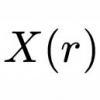### Implicitly

##### Age 16 to 18 Challenge Level:

Can you find the maximum value of the curve defined by this expression?### Resistance

##### Age 16 to 18 Challenge Level:

Find the equation from which to calculate the resistance of an infinite network of resistances.### Plus or Minus

##### Age 16 to 18 Challenge Level:

Make and prove a conjecture about the value of the product of the Fibonacci numbers $F_{n+1}F_{n-1}$.### Golden Mathematics

##### Age 16 to 18

A voyage of discovery through a sequence of challenges exploring properties of the Golden Ratio and Fibonacci numbers.### Golden Eggs

##### Age 16 to 18 Challenge Level:

Find a connection between the shape of a special ellipse and an infinite string of nested square roots.### Golden Construction

##### Age 16 to 18 Challenge Level:

Draw a square and an arc of a circle and construct the Golden rectangle. Find the value of the Golden Ratio.### Polar Flower

##### Age 16 to 18 Challenge Level:

This polar equation is a quadratic. Plot the graph given by each factor to draw the flower.### Golden Fibs

##### Age 16 to 18 Challenge Level:

When is a Fibonacci sequence also a geometric sequence? When the ratio of successive terms is the golden ratio!### Xtra

##### Age 14 to 18 Challenge Level:

Find the sides of an equilateral triangle ABC where a trapezium BCPQ is drawn with BP=CQ=2 , PQ=1 and AP+AQ=sqrt7 . Note: there are 2 possible interpretations.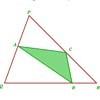### Halving the Triangle

##### Age 16 to 18 Challenge Level:

Draw any triangle PQR. Find points A, B and C, one on each side of the triangle, such that the area of triangle ABC is a given fraction of the area of triangle PQR.### Pentakite

##### Age 14 to 18 Challenge Level:

ABCDE is a regular pentagon of side length one unit. BC produced meets ED produced at F. Show that triangle CDF is congruent to triangle EDB. Find the length of BE.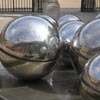### How Many Balls?

##### Age 16 to 18 Challenge Level:

A bag contains red and blue balls. You are told the probabilities of drawing certain combinations of balls. Find how many red and how many blue balls there are in the bag.### Golden Ratio

##### Age 16 to 18 Challenge Level:

Solve an equation involving the Golden Ratio phi where the unknown occurs as a power of phi.### Continued Fractions II

##### Age 16 to 18

In this article we show that every whole number can be written as a continued fraction of the form k/(1+k/(1+k/...)).### Pent

##### Age 14 to 18 Challenge Level:

The diagram shows a regular pentagon with sides of unit length. Find all the angles in the diagram. Prove that the quadrilateral shown in red is a rhombus.### Pareq Calc

##### Age 14 to 16 Challenge Level:

Triangle ABC is an equilateral triangle with three parallel lines going through the vertices. Calculate the length of the sides of the triangle if the perpendicular distances between the parallel. . . .### Cocked Hat

##### Age 16 to 18 Challenge Level:

Sketch the graphs for this implicitly defined family of functions.### Darts and Kites

##### Age 14 to 16 Challenge Level:

Explore the geometry of these dart and kite shapes!### Target Six

##### Age 16 to 18 Challenge Level:

Show that x = 1 is a solution of the equation x^(3/2) - 8x^(-3/2) = 7 and find all other solutions.### Good Approximations

##### Age 16 to 18 Challenge Level:

Solve quadratic equations and use continued fractions to find rational approximations to irrational numbers.### Kissing

##### Age 16 to 18 Challenge Level:

Two perpendicular lines are tangential to two identical circles that touch. What is the largest circle that can be placed in between the two lines and the two circles and how would you construct it?### Two Cubes

##### Age 14 to 16 Challenge Level:

Two cubes, each with integral side lengths, have a combined volume equal to the total of the lengths of their edges. How big are the cubes? [If you find a result by 'trial and error' you'll need to. . . .